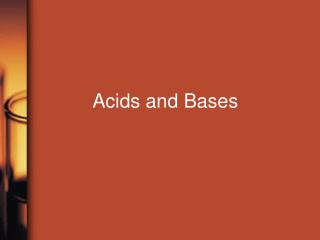DownloadDownload PresentationAcids and Bases

# Acids and Bases

Download Presentation## Acids and Bases

- - - - - - - - - - - - - - - - - - - - - - - - - - - E N D - - - - - - - - - - - - - - - - - - - - - - - - - - -
##### Presentation Transcript

1. Acids and Bases

2. Three Definitions • Arrhenius • acid – produces H+ in soln • base – produces OH- in soln • Bronsted-Lowry • acid – H+ donor • base – H+ acceptor • Lewis • acid – electron pair acceptor • base – electron pair donor

3. Conjugate acid-base pairs NH3 + H2O  NH4+ + OH- HCl + H2O  H3O+ + Cl-

4. Conjugate acid-base pairs The stronger a Bronsted { }, the weaker its conjugate { }.

5. Water . . . . . . can act as an acid or a base. So it can undergo autoionization. H2O + H2O  H3O+ + OH- K =

6. Kw = [H3O+][OH-] = 1.0 x 10-14 Neutral: [H+] [OH-] = = 1.0 x 10-7 M <

7. Kw = [H3O+][OH-] = 1.0 x 10-14 Neutral: [H+] [OH-] Acidic : [H+] [OH-] Basic : [H+] [OH-] = = 1.0 x 10-7 M > [H+] > 1.0 x 10-7 M < [OH-] 1.0 x 10-7 M 1.0 x 10-7 M [H+] < < [OH-] 1.0 x 10-7 M >

8. pH = -log [H+] pOH = -log [OH-] pH + pOH = 14.00 pH Scale

9. pH Scale pH pH pH = 7 Neutral: [H+] = [OH-] = 1.0x10-7 Acidic : [H+] > 1.0 x 10-7 M Basic : [H+] < 1.0 x 10-7 M < 7 > 7

10. pH Scale • pH = 7 Neutral: [H+] = [OH-] = 1.0x10-7 Acidic : [H+] > 1.0 x 10-7 M [OH-] < 1.0 x 10-7 M Basic : [H+] < 1.0 x 10-7 M [OH-] > 1.0 x 10-7 M • = 7 • pOH • pH < 7 • pOH > 7 pH > 7 pOH< 7

11. Determine the pOH, [OH-] and [H+] for a solution of vinegar with pH = 3.26. SIG FIGS!!!

12. Strong Acids Weak Acids only partially ionize ALL other acids • ionize completely • memorize them!!! HCl, HBr, HI, HClO4, H2SO4, HNO3

13. Strong Acids Weak Acids only partially ionize. need ICE table to calc [H+] ALL other acids weak electrolytes • ionize completely. [H+] = [HA]o • memorize them!!! HCl, HBr, HI, HClO4, H2SO4, HNO3 • strong electrolytes

14. Weak Acids • achieve equilibrium. soln mix contains mostlynonionized acid molecules, with some H3O+ and conjugate base ions.

15. Weak Acids What does the Kavalue tell us? Ka (HNO2) = 4.6 x 10-4 Ka (HC2H3O2) = 1.8 x 10-5 Ka (HCN) = 4.9 x 10-10

16. Bases • “Strong” and “weak” are used in the same way to describe bases. • memorize list of strong bases!!! LiOH, NaOH, KOH, RbOH, CsOH, Ca(OH)2*, Sr(OH)2, Ba(OH)2 *borderline

17. Bases • most common weak base is NH3 • many others are a derivative of NH3 (replace one or more hydrogens with some other group: CH3NH2)

18. Conjugate Acid-Base Pairs Ka x Kb = Kw = 1.0 x 10-14 NH3 / NH4+ Kb (NH3) = 1.8 x 10-5 Ka (NH4+) = Shows mathematically that the stronger an acid, the weaker its conjugate base.

19. Lactic acid (HC3H5O3), which is present in sour milk, also gives sauerkraut its tartness. It is a monoprotic acid. In a 0.100M solution of lactic acid, the pH is 2.44 at 25C. Calculate the Ka for lactic acid.

20. A student planned an experiment that would use 0.10M propionic acid, HC3H5O2. Calculate the values of [H+] and pH for this solution. For propionic acid, Ka = 1.4 x 10-5.

21. A solution of hydrazine, C2H4, has a concentration of 0.25M. What is the pH of the solution? Kb (C2H4) = 1.7 x 10-6.